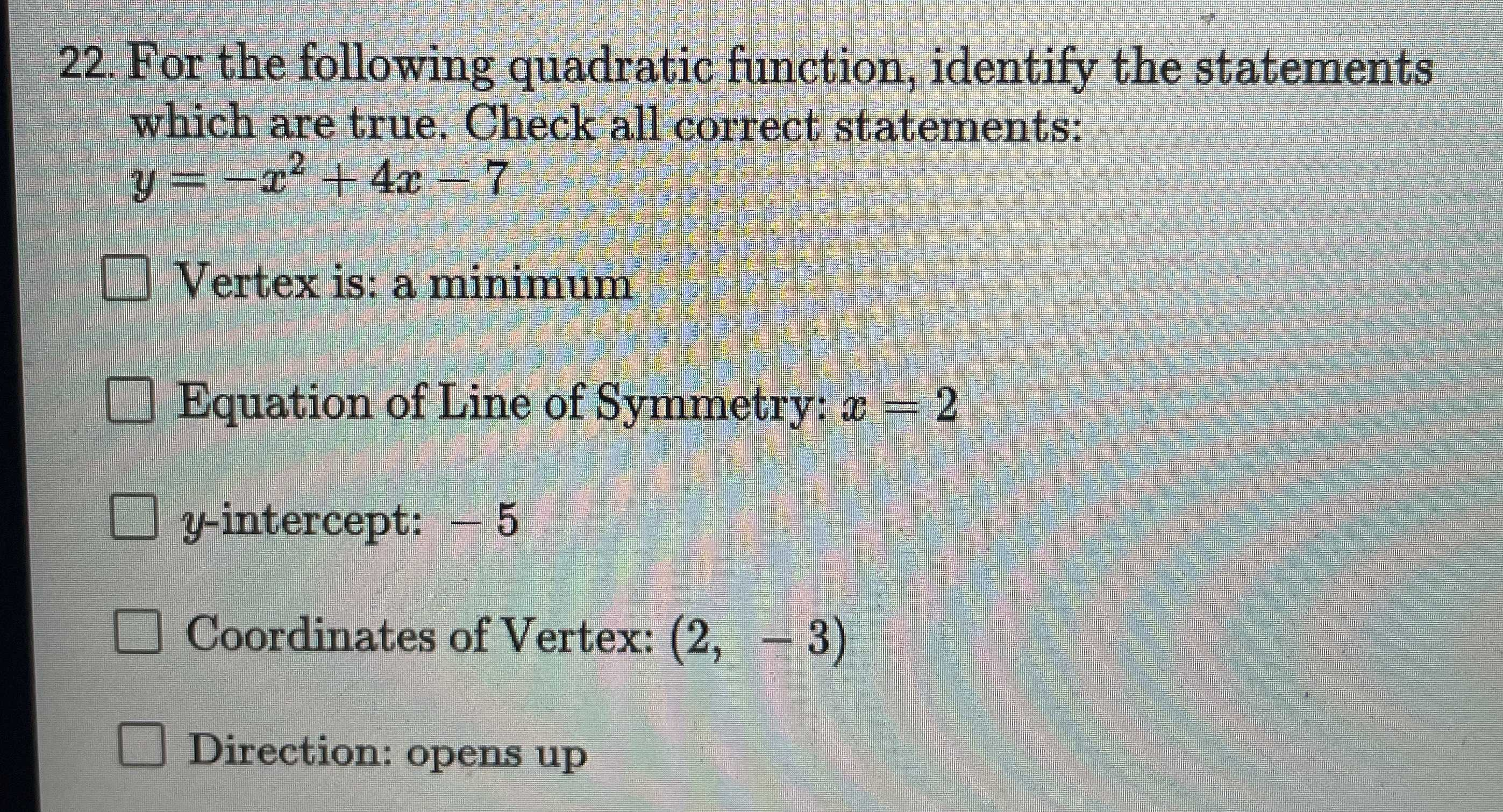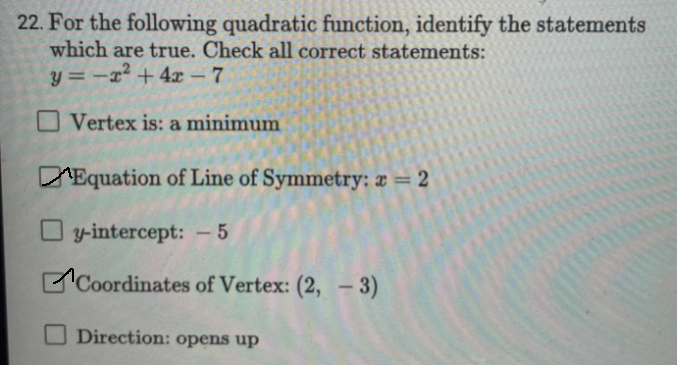### ¿Todavía tienes preguntas de matemáticas?

Pregunte a nuestros tutores expertos
Algebra
Pregunta22. For the following quadratic function, identify the statements which are true. Check all correct statements:

$$y = - x ^ { 2 } + 4 x - 7$$

$$\square$$ Vertex is: a minimum

$$\square$$ Equation of Line of Symmetry: $$x = 2$$

$$\square$$ -intercept: $$- 5$$ Coordinates of Vertex: $$( 2 , - 3 )$$

Direction: opens up